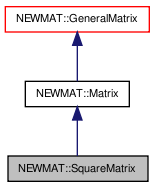# NEWMAT::SquareMatrix Class Reference

`#include <newmat.h>`

Inheritance diagram for NEWMAT::SquareMatrix:[legend]

## Detailed Description

Definition at line 604 of file newmat.h.

List of all members.

## Public Member Functions

SquareMatrix ()
~SquareMatrix ()
SquareMatrix (ArrayLengthSpecifier)
SquareMatrix (const BaseMatrix &)
void operator= (const BaseMatrix &)
SquareMatrixoperator= (Real f)
SquareMatrixoperator= (const SquareMatrix &m)
void operator= (const Matrix &m)
MatrixType Type () const
SquareMatrix (const SquareMatrix &gm)
SquareMatrix (const Matrix &gm)
void ReSize (int)
virtual void ReSize (int, int)
void ReSize (const GeneralMatrix &A)
void operator+= (const Matrix &M)
void operator-= (const Matrix &M)
void operator+= (Real f)
void operator-= (Real f)
void swap (SquareMatrix &gm)

## Private Member Functions

GeneralMatrixImage () const

## Constructor & Destructor Documentation

 NEWMAT::SquareMatrix::SquareMatrix ( )

Definition at line 608 of file newmat.h.

Referenced by Image().

 NEWMAT::SquareMatrix::~SquareMatrix ( )

Definition at line 609 of file newmat.h.

 NEWMAT::SquareMatrix::SquareMatrix ( ArrayLengthSpecifier n )

Definition at line 51 of file newmat4.cpp.

 NEWMAT::SquareMatrix::SquareMatrix ( const BaseMatrix & M )

Definition at line 78 of file newmat4.cpp.

 NEWMAT::SquareMatrix::SquareMatrix ( const SquareMatrix & gm )

Definition at line 617 of file newmat.h.

 NEWMAT::SquareMatrix::SquareMatrix ( const Matrix & gm )

Definition at line 89 of file newmat4.cpp.

## Member Function Documentation

 GeneralMatrix * NEWMAT::SquareMatrix::Image ( ) const` [private, virtual]`

Reimplemented from NEWMAT::Matrix.

Definition at line 760 of file newmat4.cpp.

 void NEWMAT::SquareMatrix::operator+= ( Real f )

Reimplemented from NEWMAT::Matrix.

Definition at line 625 of file newmat.h.

 void NEWMAT::SquareMatrix::operator+= ( const Matrix & M )

Reimplemented from NEWMAT::Matrix.

Definition at line 623 of file newmat.h.

 void NEWMAT::SquareMatrix::operator-= ( Real f )

Reimplemented from NEWMAT::Matrix.

Definition at line 626 of file newmat.h.

 void NEWMAT::SquareMatrix::operator-= ( const Matrix & M )

Reimplemented from NEWMAT::Matrix.

Definition at line 624 of file newmat.h.

 void NEWMAT::SquareMatrix::operator= ( const Matrix & m )

Reimplemented from NEWMAT::Matrix.

Definition at line 361 of file newmat6.cpp.

 SquareMatrix& NEWMAT::SquareMatrix::operator= ( const SquareMatrix & m )

Definition at line 614 of file newmat.h.

 SquareMatrix& NEWMAT::SquareMatrix::operator= ( Real f )

Reimplemented from NEWMAT::Matrix.

Definition at line 613 of file newmat.h.

 void NEWMAT::SquareMatrix::operator= ( const BaseMatrix & X )

Reimplemented from NEWMAT::Matrix.

Definition at line 352 of file newmat6.cpp.

Referenced by operator=().

 void NEWMAT::SquareMatrix::ReSize ( const GeneralMatrix & A ) ` [virtual]`

Reimplemented from NEWMAT::Matrix.

Definition at line 299 of file newmat4.cpp.

 void NEWMAT::SquareMatrix::ReSize ( int nr, int nc ) ` [virtual]`

Reimplemented from NEWMAT::Matrix.

Definition at line 252 of file newmat4.cpp.

 void NEWMAT::SquareMatrix::ReSize ( int n )

Definition at line 249 of file newmat4.cpp.

Referenced by ReSize().

 void NEWMAT::SquareMatrix::swap ( SquareMatrix & gm )

Definition at line 627 of file newmat.h.

Referenced by NEWMAT::swap().

 MatrixType NEWMAT::SquareMatrix::Type ( ) const` [virtual]`

Reimplemented from NEWMAT::Matrix.

Definition at line 427 of file newmat4.cpp.

The documentation for this class was generated from the following files:

 newmat11b Generated Mon May 9 04:54:19 2016 by Doxygen 1.6.3